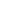# Free CBSE Class 8 Worksheets

Download free printable Class 8 Worksheets to practice. With thousands of questions available, you can generate as many Class 8 Worksheets as you want.

## Sample CBSE Class 8 Worksheet Questions

1.

A car travels at a speed of 40 kmph for the onward journey and 50 kmph for the return journey. What is the average speed ?

1.

44.44

2.

45

3.

44

4.

45.44

2.

A person rows a kilometre down the stream in 10 minutes and up the stream in 30 minutes. Find the velocity of the stream.

1.

2

2.

3

3.

4

4.

5

3.

A monkey climbing a greased pole ascends 12 m and slips down 5 m. If the pole is 63 m high, how long will it take for the monkey to reaach the top

1.

16 min 35 sec

2.

16 min

3.

17 min 35 s

4.

17 min

4.

In an army there are 840 soldiers. They have enough food for 70 days. After 5 days 210 soldiers join the army. Find the no. of days for which the remaining food will be sufficient

1.

40

2.

55

3.

52

4.

60

5.

A gardener planted 103041 trees in such a way that the number of rows were as many as were the trees in a row. Find the no. of rows

1.

321

2.

320

3.

319

4.

221

6.

Sum of angles around a point

1.

90 deg

2.

180 deg

3.

270 deg

4.

360 deg

7.

A man purchases 5 horses and 10 cows for Rs 10000. He sells the horses at 15% profit and cows at 10% loss. Thus he gets Rs 375 as profit. Find the individual cost of 1 horse and 1 cow.

1.

Let CP of horse = Rs x and CP of cow = Rs y

5x + 10y = 10000

Horses profit = 15% = Rs 15x/100 * 5 and Cows loss = 10% = 10y/100 * 10

So 15x/100 - 10y/100 = 375

Solving the above 2 equations we get, CP of 1 horse is Rs 1100 and CP of 1 cow is Rs 450

2.

Refer above

8.

Cost Price of 12 pens is equal to Selling Price of 10 pens. Find the gain or loss percent

1.

Let CP of 12 pens = Rs 100

So SP of 10 pens = Rs 100. Therefore SP of 12 pens = 12*100/10 = Rs 120

Gain = Rs 120 - Rs 100 = Rs 20. So Gain percent is 20

2.

Refer above

9.

In an examination, 40% failed in Maths and 30% failed in English. 20% failed in both the subjects. Find the pass percentage.

1.

Let total no. of students be 100. So 40 students failed in Maths, 30 students failed in English and 20 students failed in both the subjects. Using the concept of Venn Diagrams shown in the below image, we get total no. of failed students as 20+20+10 = 50 students.

So the no. of students who have passed is 100 - 50 = 50 students. So pass % is 50%2.

Refer above

10.

A's salary is 25% more than that of B. By how much is B's salary lower when compared to A ?

1.

Let B's salary = Rs 100

A's salary = 25% more than B = 25/100*100 = Rs 25

So A's salary = 100 +25 = Rs 125

if A's salary is Rs 125, B's salary is Rs 100

So if A's salary is Rs 100, then B' salary = 100*100/125 = Rs 80

So B's salary is Rs 20 less than that of A i.e B's salary is 20% less than that of A

2.

Refer above

## Find more Class 8 Worksheets

Worksheets by UrbanPro

Our worksheets are designed to help students explore various topics, practice skills and enrich their subject knowledge, to improve their academic performance. Designed by Experts who have extensive experience and expertise in teaching a subject, these worksheets will improve your child's problem-solving skills and subject knowledge in a fun and interactive manner.
Check out our free customized worksheets across school boards, grades, subjects and levels of subject knowledge. You can download, print and share these worksheets with anyone, anywhere, anytime!

Get a custom worksheet to practice!

Select your topic & see the magic.

subjectSelect a subject*

Maths Science Social Studies

Select Chapter(s)

Chapters & Subtopics